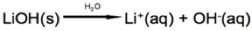# Arrhenius Concept of Acids and Bases

$$\newcommand{\vecs}{\overset { \rightharpoonup} {\mathbf{#1}} }$$ $$\newcommand{\vecd}{\overset{-\!-\!\rightharpoonup}{\vphantom{a}\smash {#1}}}$$$$\newcommand{\id}{\mathrm{id}}$$ $$\newcommand{\Span}{\mathrm{span}}$$ $$\newcommand{\kernel}{\mathrm{null}\,}$$ $$\newcommand{\range}{\mathrm{range}\,}$$ $$\newcommand{\RealPart}{\mathrm{Re}}$$ $$\newcommand{\ImaginaryPart}{\mathrm{Im}}$$ $$\newcommand{\Argument}{\mathrm{Arg}}$$ $$\newcommand{\norm}{\| #1 \|}$$ $$\newcommand{\inner}{\langle #1, #2 \rangle}$$ $$\newcommand{\Span}{\mathrm{span}}$$ $$\newcommand{\id}{\mathrm{id}}$$ $$\newcommand{\Span}{\mathrm{span}}$$ $$\newcommand{\kernel}{\mathrm{null}\,}$$ $$\newcommand{\range}{\mathrm{range}\,}$$ $$\newcommand{\RealPart}{\mathrm{Re}}$$ $$\newcommand{\ImaginaryPart}{\mathrm{Im}}$$ $$\newcommand{\Argument}{\mathrm{Arg}}$$ $$\newcommand{\norm}{\| #1 \|}$$ $$\newcommand{\inner}{\langle #1, #2 \rangle}$$ $$\newcommand{\Span}{\mathrm{span}}$$$$\newcommand{\AA}{\unicode[.8,0]{x212B}}$$

The Arrhenius definition can be summarized as "Arrhenius acids form hydrogen ions in aqueous solution with Arrhenius bases forming hydroxide ions."

## Introduction

In 1884, the Swedish chemist Svante Arrhenius proposed two specific classifications of compounds, termed acids and bases. When dissolved in an aqueous solution, certain ions were released into the solution. As defined by Arrhenius, acid-base reactions are characterized by acids, which dissociate in aqueous solution to form hydrogen ions (H+) and bases, which form hydroxide (OH) ions.

Acids are defined as a compound or element that releases hydrogen (H+) ions into the solution (mainly water).

$NHO_3 (aq) + H_2O(l) \rightarrow H_3O^+ + NO_3^- (aq)$

In this reaction nitric acid (HNO3) disassociates into hydrogen (H+) and nitrate (NO3-) ions when dissolved in water. Bases are defined as a compound or element that releases hydroxide (OH-) ions into the solution.In this reaction lithium hydroxide (LiOH) dissociates into lithium (Li+) and hydroxide (OH-) ions when dissolved in water.

Arrhenius Concept of Acids and Bases is shared under a CC BY-NC-SA 4.0 license and was authored, remixed, and/or curated by LibreTexts.